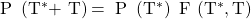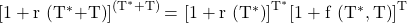Essential Concept 62: Forward Pricing and Forward Rate Models | IFT World
101 Concepts for the Level I Exam

# Essential Concept 62: Forward Pricing and Forward Rate ModelsForward pricing model: It is expressed as:If T* is 1 and T is 2, the present value of \$1 to be received 3 years from today, P(3), is given by P(3) = P(1)F(1, 2).

Forward rate model: If we express the forward pricing model in terms of rates, we get the forward rate model.If T* is 1 and T is 2, then (1 + r(3))3 = (1 + r(1))1(1 + f(1,2))2

Two interpretations of forward rates: Suppose f(1,2), the rate agreed on today for a two-year loan to be made one year from today, is 12%.

• Interpretation 1: 12% is the reinvestment rate that would make an investor indifferent between buying a three-year zero-coupon bond or investing in a one-year zero-coupon bond and at maturity reinvesting the proceeds for two years. In this sense, the forward rate can be viewed as a type of breakeven interest rate.
• Interpretation 2: 12% is the two-year rate that can be locked in today by buying a three-year zero-coupon bond rather than investing in a one-year zero-coupon bond and, when it matures, reinvesting the proceeds in a zero-coupon instrument that matures in two years. In this sense, the forward rate can be viewed as a rate that can be locked in by extending maturity by two years.

Implication of the forward rate model

• If the spot curve is upward sloping then the forward curve lies above the spot curve.
• If the spot curve is downward sloping then the forward curve lies below the spot curve.